Anzeige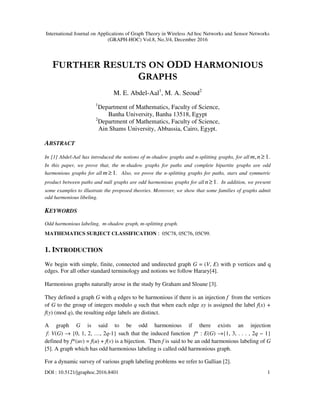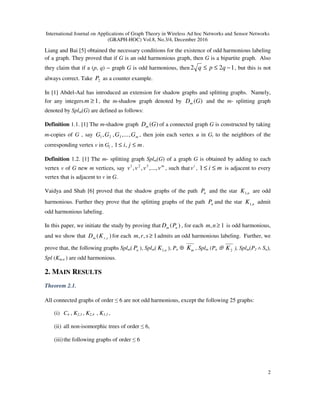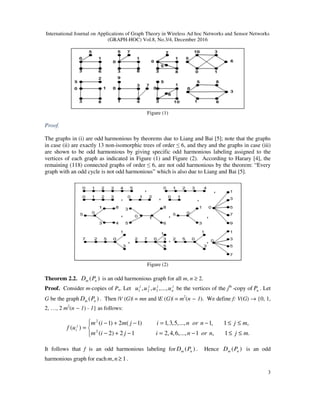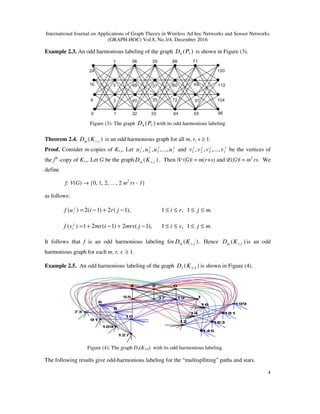Anzeige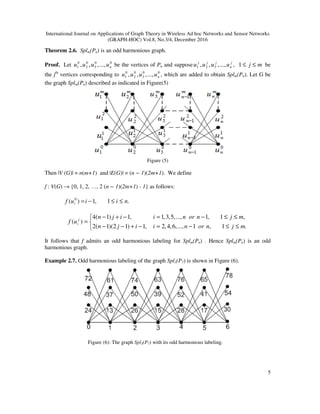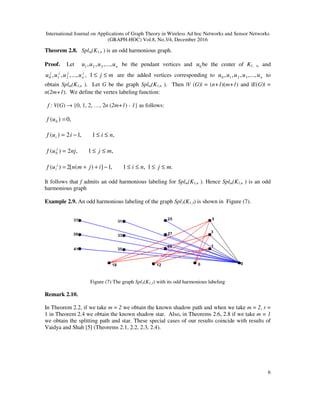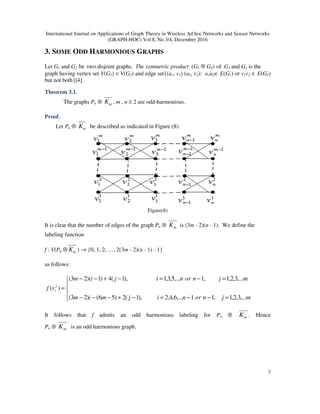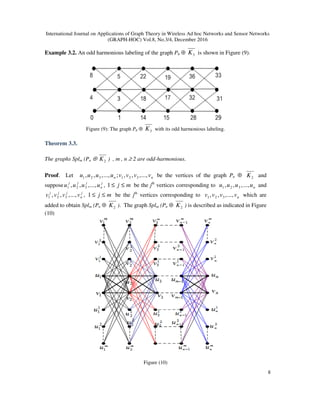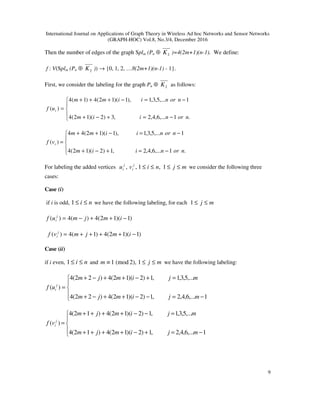Anzeige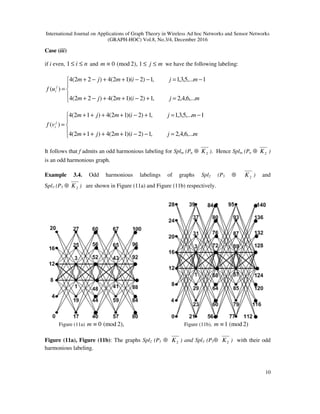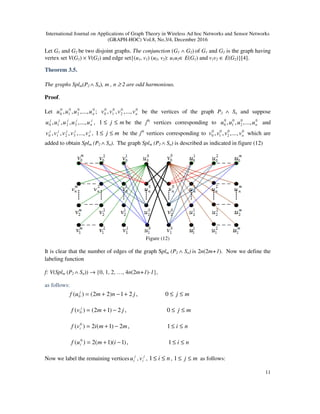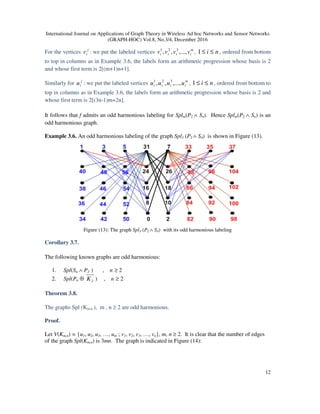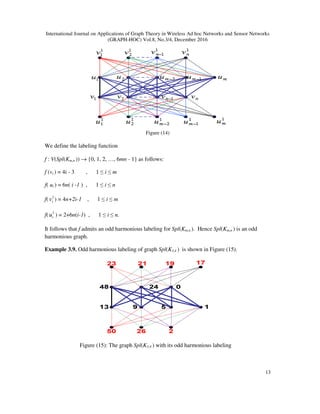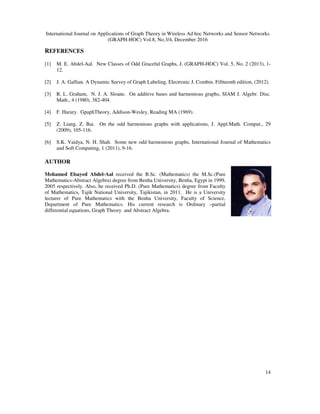Nächste SlideShare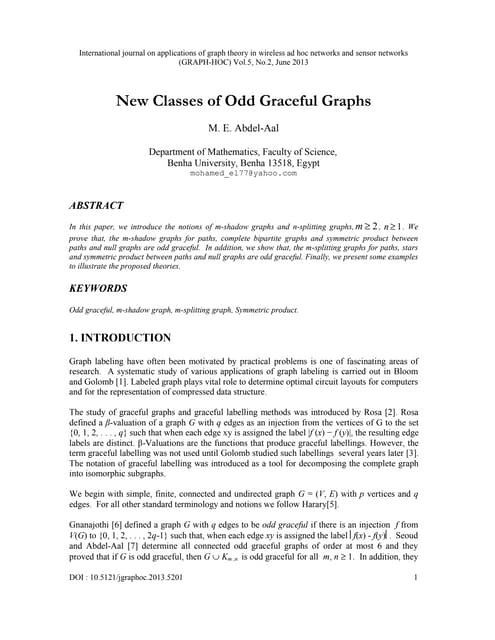New Classes of Odd Graceful Graphs
1 von 14
Anzeige

### FURTHER RESULTS ON ODD HARMONIOUS GRAPHS

1. International Journal on Applications of Graph Theory in Wireless Ad hoc Networks and Sensor Networks (GRAPH-HOC) Vol.8, No.3/4, December 2016 DOI : 10.5121/jgraphoc.2016.8401 1 FURTHER RESULTS ON ODD HARMONIOUS GRAPHS M. E. Abdel-Aal1 , M. A. Seoud2 1 Department of Mathematics, Faculty of Science, Banha University, Banha 13518, Egypt 2 Department of Mathematics, Faculty of Science, Ain Shams University, Abbassia, Cairo, Egypt. ABSTRACT In  Abdel-Aal has introduced the notions of m-shadow graphs and n-splitting graphs, for all 1, ≥nm . In this paper, we prove that, the m-shadow graphs for paths and complete bipartite graphs are odd harmonious graphs for all 1≥m . Also, we prove the n-splitting graphs for paths, stars and symmetric product between paths and null graphs are odd harmonious graphs for all 1≥n . In addition, we present some examples to illustrate the proposed theories. Moreover, we show that some families of graphs admit odd harmonious libeling. KEYWORDS Odd harmonious labeling, m-shadow graph, m-splitting graph. MATHEMATICS SUBJECT CLASSIFICATION : 05C78, 05C76, 05C99. 1. INTRODUCTION We begin with simple, finite, connected and undirected graph G = (V, E) with p vertices and q edges. For all other standard terminology and notions we follow Harary. Harmonious graphs naturally arose in the study by Graham and Sloane . They defined a graph G with q edges to be harmonious if there is an injection f from the vertices of G to the group of integers modulo q such that when each edge xy is assigned the label f(x) + f(y) (mod q), the resulting edge labels are distinct. A graph G is said to be odd harmonious if there exists an injection f: V(G) → {0, 1, 2, …, 2q-1} such that the induced function f* : E(G) →{1, 3, . . . , 2q − 1} defined by f*(uv) = f(u) + f(v) is a bijection. Then f is said to be an odd harmonious labeling of G . A graph which has odd harmonious labeling is called odd harmonious graph. For a dynamic survey of various graph labeling problems we refer to Gallian .
2. International Journal on Applications of Graph Theory in Wireless Ad hoc Networks and Sensor Networks (GRAPH-HOC) Vol.8, No.3/4, December 2016 2 Liang and Bai  obtained the necessary conditions for the existence of odd harmonious labeling of a graph. They proved that if G is an odd harmonious graph, then G is a bipartite graph. Also they claim that if a (p, q) − graph G is odd harmonious, then 122 −≤≤ qpq , but this is not always correct. Take 2P as a counter example. In  Abdel-Aal has introduced an extension for shadow graphs and splitting graphs. Namely, for any integers 1≥m , the m-shadow graph denoted by )(GDm and the m- splitting graph denoted by Splm(G) are defined as follows: Definition 1.1.  The m-shadow graph )(GDm of a connected graph G is constructed by taking m-copies of G , say mGGGG ,...,,, 321 , then join each vertex u in Gi to the neighbors of the corresponding vertex v in Gj , mji ≤≤ ,1 . Definition 1.2.  The m- splitting graph Splm(G) of a graph G is obtained by adding to each vertex v of G new m vertices, say m vvvv ...,,,, 321 , such that i v , mi ≤≤1 is adjacent to every vertex that is adjacent to v in G. Vaidya and Shah  proved that the shadow graphs of the path nP and the star nK ,1 are odd harmonious. Further they prove that the splitting graphs of the path nP and the star nK ,1 admit odd harmonious labeling. In this paper, we initiate the study by proving that )( nm PD , for each 1, ≥nm is odd harmonious, and we show that )( ,srm KD for each 1,, ≥srm admits an odd harmonious labeling. Further, we prove that, the following graphs Splm( nP ), Splm( nK ,1 ), Pn ⊕ mK , Splm (Pn ⊕ 2K ), Splm(P2 ∧ Sn), Spl (Km,n ) are odd harmonious. 2. MAIN RESULTS Theorem 2.1. All connected graphs of order ≤ 6 are not odd harmonious, except the following 25 graphs: (i) C4 , K2,3 , K2,4 , K3,3 , (ii) all non-isomorphic trees of order ≤ 6, (iii)the following graphs of order ≤ 6
3. International Journal on Applications of Graph Theory in Wireless Ad hoc Networks and Sensor Networks (GRAPH-HOC) Vol.8, No.3/4, December 2016 3 Figure (1) Proof. The graphs in (i) are odd harmonious by theorems due to Liang and Bai ; note that the graphs in case (ii) are exactly 13 non-isomorphic trees of order ≤ 6, and they and the graphs in case (iii) are shown to be odd harmonious by giving specific odd harmonious labeling assigned to the vertices of each graph as indicated in Figure (1) and Figure (2). According to Harary , the remaining (118) connected graphs of order ≤ 6, are not odd harmonious by the theorem: “Every graph with an odd cycle is not odd harmonious” which is also due to Liang and Bai . Figure (2) Theorem 2.2. )( nm PD is an odd harmonious graph for all m, n ≥ 2. Proof. Consider m-copies of Pn. Let j n jjj uuuu ...,,,, 321 be the vertices of the jth -copy of nP . Let G be the graph )( nm PD . Then |V (G)| = mn and |E (G)| = m2 (n − 1). We define f: V(G) → {0, 1, 2, …, 2 m2 (n − 1) - 1} as follows:     ≤≤−=−+− ≤≤−=−+− = .1,1...,,6,4,212)2( ,1,1...,,5,3,1)1(2)1( )( 2 2 mjnornijim mjnornijmim uf j i It follows that f is an odd harmonious labeling for )( nm PD . Hence )( nm PD is an odd harmonious graph for each 1, ≥nm .
4. International Journal on Applications of Graph Theory in Wireless Ad hoc Networks and Sensor Networks (GRAPH-HOC) Vol.8, No.3/4, December 2016 4 Example 2.3. An odd harmonious labeling of the graph )( 74 PD is shown in Figure (3). Figure (3): The graph )( 74 PD with its odd harmonious labeling Theorem 2.4. )( ,srm KD is an odd harmonious graph for all m, r, s ≥ 1. Proof. Consider m-copies of Kr,s. Let j r jjj uuuu ...,,,, 321 and j s jjj vvvv ...,,,, 321 be the vertices of the jth -copy of Kr,s. Let G be the graph )( ,srm KD . Then |V (G)| = m(r+s) and |E(G)| = m2 rs. We define f: V(G) → {0, 1, 2, …, 2 m2 rs - 1} as follows: .1,1),1(2)1(2)( mjrijriuf j i ≤≤≤≤−+−= .1,1),1(2)1(21)( mjsijmrsimrvf j i ≤≤≤≤−+−+= It follows that f is an odd harmonious labeling for )( ,srm KD . Hence )( ,srm KD is an odd harmonious graph for each m, r, s ≥ 1. Example 2.5. An odd harmonious labeling of the graph )( 4,33 KD is shown in Figure (4). Figure (4): The graph D3(K3,4) with its odd harmonious labeling. The following results give odd-harmonious labeling for the “multisplliting” paths and stars.
5. International Journal on Applications of Graph Theory in Wireless Ad hoc Networks and Sensor Networks (GRAPH-HOC) Vol.8, No.3/4, December 2016 5 Theorem 2.6. Splm(Pn) is an odd harmonious graph. Proof. Let 00 3 0 2 0 1 ...,,,, nuuuu be the vertices of Pn and suppose j n jjj uuuu ...,,,, 321 , mj ≤≤1 be the jth vertices corresponding to 00 3 0 2 0 1 ...,,,, nuuuu , which are added to obtain Splm(Pn). Let G be the graph Splm(Pn) described as indicated in Figure(5) Figure (5) Then |V (G)| = n(m+1) and |E(G)| = (n − 1)(2m+1). We define f : V(G) → {0, 1, 2, …, 2 (n − 1)(2m+1) - 1} as follows: .1,1)( 0 niiuf i ≤≤−=    ≤≤−=−+−− ≤≤−=−+− = .1,1...,,6,4,2,1)12)(1(2 ,1,1...,,5,3,1,1)1(4 )( mjnorniijn mjnorniijn uf j i It follows that f admits an odd harmonious labeling for Splm(Pn) . Hence Splm(Pn) is an odd harmonious graph. Example 2.7. Odd harmonious labeling of the graph Spl3(P7) is shown in Figure (6). Figure (6): The graph Spl3(P7) with its odd harmonious labeling.
6. International Journal on Applications of Graph Theory in Wireless Ad hoc Networks and Sensor Networks (GRAPH-HOC) Vol.8, No.3/4, December 2016 6 Theorem 2.8. Splm(K1,n ) is an odd harmonious graph. Proof. Let nuuuu ...,,,, 321 be the pendant vertices and 0u be the center of K1, n, and j n jjj uuuu ...,,,, 210 , mj ≤≤1 are the added vertices corresponding to nuuuuu ...,,,,, 3210 to obtain Splm(K1,n ). Let G be the graph Splm(K1,n ). Then |V (G)| = (n+1)(m+1) and |E(G)| = n(2m+1). We define the vertex labeling function: f : V(G) → {0, 1, 2, …, 2n (2m+1) - 1} as follows: ,0)( 0 =uf ,1,12)( niiuf i ≤≤−= ,1,2)( 0 mjnjuf j ≤≤= .1,1,1])([2)( mjniijmnuf j i ≤≤≤≤−++= It follows that f admits an odd harmonious labeling for Splm(K1,n ). Hence Splm(K1,n ) is an odd harmonious graph Example 2.9. An odd harmonious labeling of the graph Spl3(K1,3) is shown in Figure (7). Figure (7) The graph Spl3(K1,3) with its odd harmonious labeling Remark 2.10. In Theorem 2.2, if we take m = 2 we obtain the known shadow path and when we take m = 2, r = 1 in Theorem 2.4 we obtain the known shadow star. Also, in Theorems 2.6, 2.8 if we take m = 1 we obtain the splitting path and star. These special cases of our results coincide with results of Vaidya and Shah  (Theorems 2.1, 2.2, 2.3, 2.4).
7. International Journal on Applications of Graph Theory in Wireless Ad hoc Networks and Sensor Networks (GRAPH-HOC) Vol.8, No.3/4, December 2016 7 3. SOME ODD HARMONIOUS GRAPHS Let G1 and G2 be two disjoint graphs. The symmetric product (G1 ⊕ G2) of G1 and G2 is the graph having vertex set V(G1) × V(G2) and edge set{(u1, v1) (u2, v2): u1u2∈ E(G1) or v1v2 ∈ E(G2) but not both}. Theorem 3.1. The graphs Pn ⊕ mK , m , n ≥ 2 are odd-harmonious. Proof. Let Pn ⊕ mK be described as indicated in Figure (8): Figure(8) It is clear that the number of edges of the graph Pn ⊕ mK is (3m - 2)(n - 1). We define the labeling function f : V(Pn ⊕ mK ) → {0, 1, 2, …, 2(3m - 2)(n - 1) - 1} as follows:      =−−=−+−−− =−=−+−− = mjnornijmim mjnornijim vf j i ,...3,2,1,11,...6,4,2),1(2)56()23( ,...3,2,1,1,...5,3,1),1(4)1)(23( )( It follows that f admits an odd harmonious labeling for Pn ⊕ mK . Hence Pn ⊕ mK is an odd harmonious graph.
8. International Journal on Applications of Graph Theory in Wireless Ad hoc Networks and Sensor Networks (GRAPH-HOC) Vol.8, No.3/4, December 2016 8 Example 3.2. An odd harmonious labeling of the graph P6 ⊕ 3K is shown in Figure (9). Figure (9): The graph P6 ⊕ 3K with its odd harmonious labeling. Theorem 3.3. The graphs Splm (Pn ⊕ 2K ) , m , n ≥ 2 are odd-harmonious. Proof. Let nn vvvvuuuu ...,,,,;...,,,, 321321 be the vertices of the graph Pn ⊕ and suppose j n jjj uuuu ...,,,, 321 , mj ≤≤1 be the jth vertices corresponding to nuuuu ...,,,, 321 and j n jjj vvvv ...,,,, 321 , mj ≤≤1 be the jth vertices corresponding to which are added to obtain Splm (Pn ⊕ 2K ). The graph Splm (Pn ⊕ 2K ) is described as indicated in Figure (10) Figure (10) 2K nvvvv ...,,,, 321
9. International Journal on Applications of Graph Theory in Wireless Ad hoc Networks and Sensor Networks (GRAPH-HOC) Vol.8, No.3/4, December 2016 9 Then the number of edges of the graph Splm (Pn ⊕ 2K )=4(2m+1)(n-1). We define: f : V(Splm (Pn ⊕ 2K )) → {0, 1, 2, …8(2m+1)(n-1) - 1}. First, we consider the labeling for the graph Pn ⊕ 2K as follows:      −=+−+ −=−+++ = .1,...6,4,2,3)2)(12(4 1,...5,3,1),1)(12(4)1(4 )( norniim norniimm uf i      −=+−+ −=−++ = .1,...6,4,2,1)2)(12(4 1,...5,3,1),1)(12(44 )( norniim norniimm vf i For labeling the added vertices mjnivu j i j i ≤≤≤≤ 1,1,, we consider the following three cases: Case (i) if i is odd, ni ≤≤1 we have the following labeling, for each mj ≤≤1 )1)(12(4)(4)( −++−= imjmuf j i )1)(12(4)1(4)( −++++= imjmvf j i Case (ii) if i even, ni ≤≤1 and ),2(mod1≡m mj ≤≤1 we have the following labeling:      −=−−++−+ =+−++−+ = 1,...6,4,2,1)2)(12(4)22(4 ,...5,3,1,1)2)(12(4)22(4 )( mjimjm mjimjm uf j i      −=+−++++ =−−++++ = 1,...6,4,2,1)2)(12(4)12(4 ,...5,3,1,1)2)(12(4)12(4 )( mjimjm mjimjm vf j i
10. International Journal on Applications of Graph Theory in Wireless Ad hoc Networks and Sensor Networks (GRAPH-HOC) Vol.8, No.3/4, December 2016 10 Case (iii) if i even, ni ≤≤1 and ),2(mod0≡m mj ≤≤1 we have the following labeling:      =+−++−+ −=−−++−+ = mjimjm mjimjm uf j i ,...6,4,2,1)2)(12(4)22(4 1,...5,3,1,1)2)(12(4)22(4 )(      =−−++++ −=+−++++ = mjimjm mjimjm vf j i ,...6,4,2,1)2)(12(4)12(4 1,...5,3,1,1)2)(12(4)12(4 )( It follows that f admits an odd harmonious labeling for Splm (Pn ⊕ 2K ). Hence Splm (Pn ⊕ 2K ) is an odd harmonious graph. Example 3.4. Odd harmonious labelings of graphs Spl2 (P5 ⊕ ) and Spl3 (P5 ⊕ 2K ) are shown in Figure (11a) and Figure (11b) respectively. Figure (11a) ),2(mod0≡m Figure (11b), )2(mod1≡m Figure (11a), Figure (11b): The graphs Spl2 (P5 ⊕ ) and Spl3 (P5⊕ ) with their odd harmonious labeling. 2K 2K 2K
11. International Journal on Applications of Graph Theory in Wireless Ad hoc Networks and Sensor Networks (GRAPH-HOC) Vol.8, No.3/4, December 2016 11 Let G1 and G2 be two disjoint graphs. The conjunction (G1 ∧ G2) of G1 and G2 is the graph having vertex set V(G1) × V(G2) and edge set{(u1, v1) (u2, v2): u1u2∈ E(G1) and v1v2 ∈ E(G2)}. Theorem 3.5. The graphs Splm(P2 ∧ Sn), m , n ≥ 2 are odd harmonious. Proof. Let 00 2 0 1 0 0 00 2 0 1 0 0 ...,,,,;...,,,, nn vvvvuuuu be the vertices of the graph P2 ∧ Sn and suppose j n jjjj uuuuu ...,,,,, 3210 , mj ≤≤1 be the jth vertices corresponding to 00 2 0 1 0 0 ...,,,, nuuuu and j n jjjj vvvvv ...,,,,, 3210 , mj ≤≤1 be the jth vertices corresponding to 00 2 0 1 0 0 ...,,,, nvvvv which are added to obtain Splm (P2 ∧ Sn). The graph Splm (P2 ∧ Sn) is described as indicated in figure (12) Figure (12) It is clear that the number of edges of the graph Splm (P2 ∧ Sn) is 2n(2m+1). Now we define the labeling function f: V(Splm (P2 ∧ Sn)) → {0, 1, 2, …, 4n(2m+1)-1}, as follows: mjjnmuf j ≤≤+−+= 0,21)22()( 0 mjjmvf j ≤≤−+= 0,2)12()( 0 nimmivf i ≤≤−+= 1,2)1(2)( 0 niimuf i ≤≤−+= 1,)1)(1(2)( 0 Now we label the remaining vertices j iu , j iv , ni ≤≤1 , mj ≤≤1 as follows:
12. International Journal on Applications of Graph Theory in Wireless Ad hoc Networks and Sensor Networks (GRAPH-HOC) Vol.8, No.3/4, December 2016 12 For the vertices j iv : we put the labeled vertices m iiii vvvv ,...,,, 321 , ni ≤≤1 , ordered from bottom to top in columns as in Example 3.6, the labels form an arithmetic progression whose basis is 2 and whose first term is 2[(m+1)n+1]. Similarly for j iu : we put the labeled vertices m iiii uuuu ,...,,, 321 , ni ≤≤1 , ordered from bottom to top in columns as in Example 3.6, the labels form an arithmetic progression whose basis is 2 and whose first term is 2[(3n-1)m+2n]. It follows that f admits an odd harmonious labeling for Splm(P2 ∧ Sn). Hence Splm(P2 ∧ Sn) is an odd harmonious graph. Example 3.6. An odd harmonious labeling of the graph Spl3 (P2 ∧ S4) is shown in Figure (13). Figure (13): The graph Spl3 (P2 ∧ S4) with its odd harmonious labeling Corollary 3.7. The following known graphs are odd harmonious: 1. Spl(Sn ∧ P2 ) , n ≥ 2 2. Spl(Pn ⊕ 2K ) , n ≥ 2 Theorem 3.8. The graphs Spl (Km,n ), m , n ≥ 2 are odd harmonious. Proof. Let V(Km,n) = {u1, u2, u3, …, um ; v1, v2, v3, …, vn}, m, n ≥ 2. It is clear that the number of edges of the graph Spl(Km,n) is 3mn. The graph is indicated in Figure (14):
13. International Journal on Applications of Graph Theory in Wireless Ad hoc Networks and Sensor Networks (GRAPH-HOC) Vol.8, No.3/4, December 2016 13 Figure (14) We define the labeling function f : V(Spl(Km,n )) → {0, 1, 2, …, 6mn - 1} as follows: f (vi ) = 4i - 3 , 1 ≤ i ≤ m f( ui ) = 6n( i -1 ) , 1 ≤ i ≤ n f( ) = 4n+2i-1 , 1 ≤ i ≤ m f( ) = 2+6n(i-1) , 1 ≤ i ≤ n. It follows that f admits an odd harmonious labeling for Spl(Km,n ). Hence Spl(Km,n ) is an odd harmonious graph. Example 3.9. Odd harmonious labeling of graph Spl(K3,4 ) is shown in Figure (15). Figure (15): The graph Spl(K3,4 ) with its odd harmonious labeling 1 iv 1 iu
14. International Journal on Applications of Graph Theory in Wireless Ad hoc Networks and Sensor Networks (GRAPH-HOC) Vol.8, No.3/4, December 2016 14 REFERENCES  M. E. Abdel-Aal. New Classes of Odd Graceful Graphs, J. (GRAPH-HOC) Vol. 5, No. 2 (2013), 1- 12.  J. A. Gallian. A Dynamic Survey of Graph Labeling, Electronic J. Combin. Fiftteenth edition, (2012).  R. L. Graham, N. J. A. Sloane. On additive bases and harmonious graphs, SIAM J. Algebr. Disc. Math., 4 (1980), 382-404.  F. Harary. GpaphTheory, Addison-Wesley, Reading MA (1969).  Z. Liang, Z. Bai. On the odd harmonious graphs with applications, J. Appl.Math. Comput., 29 (2009), 105-116.  S.K. Vaidya, N. H. Shah. Some new odd harmonious graphs, International Journal of Mathematics and Soft Computing, 1 (2011), 9-16. AUTHOR Mohamed Elsayed Abdel-Aal received the B.Sc. (Mathematics) the M.Sc.(Pure Mathematics-Abstract Algebra) degree from Benha University, Benha, Egypt in 1999, 2005 respectively. Also, he received Ph.D. (Pure Mathematics) degree from Faculty of Mathematics, Tajik National University, Tajikistan, in 2011. He is a University lecturer of Pure Mathematics with the Benha University, Faculty of Science, Department of Pure Mathematics. His current research is Ordinary –partial differential equations, Graph Theory and Abstract Algebra.
Anzeige